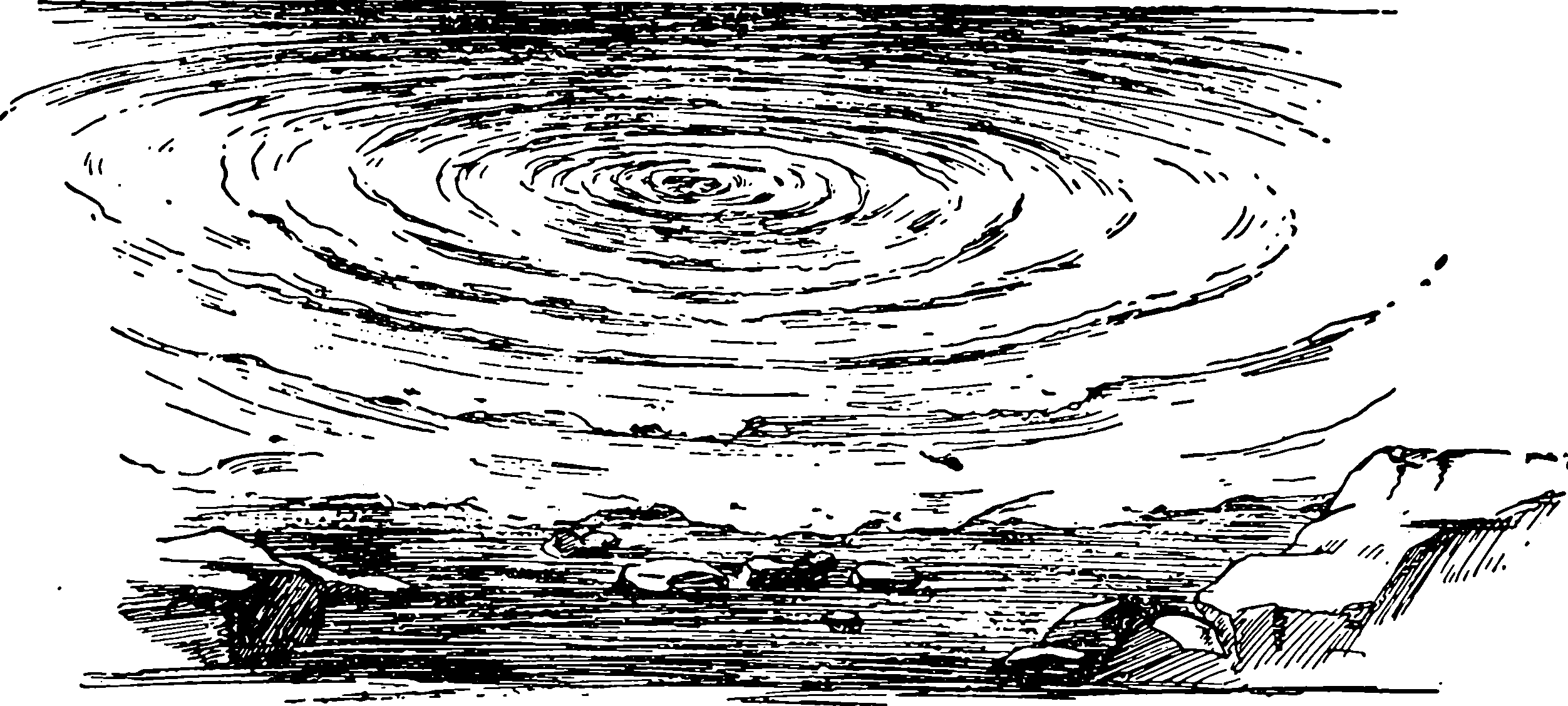# Integral transforms

$\renewcommand{\vv}{\boldsymbol{#1}} \renewcommand{\mm}{\mathrm{#1}} \renewcommand{\mmm}{\mathrm{#1}} \renewcommand{\cc}{\mathcal{#1}} \renewcommand{\ff}{\mathfrak{#1}} \renewcommand{\oo}{\operatorname{#1}} \renewcommand{\cc}{\mathcal{#1}}$

Useful for solving various integral equations and PDEs. Fourier transforms, Laplace transforms, Mellin transforms, Hankel transforms…TBD.

## Hankel transformTBD. Covered implicitly in rotational symmetries.

## Mellin transform

A transform with “Scale invariance like the Fourier transform has shift invariance”. Sounds fun.

### No comments yet. Why not leave one?

GitHub-flavored Markdown & a sane subset of HTML is supported.